##### Introduction

I would like to share how to create Extension method in c# and why we need to

have this method in C#.

First we will discuss the problem and then will rectify the problem using Extension

method.

##### Understanding the problem

1.       Add Class library as per below screen. We created Calculator library.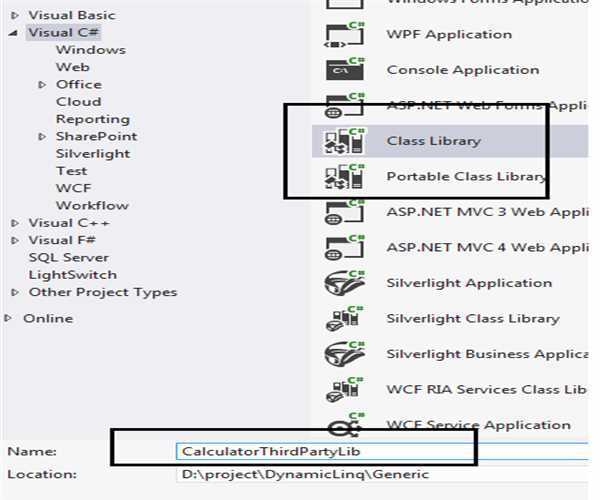2.       Add calculator class as per screen below.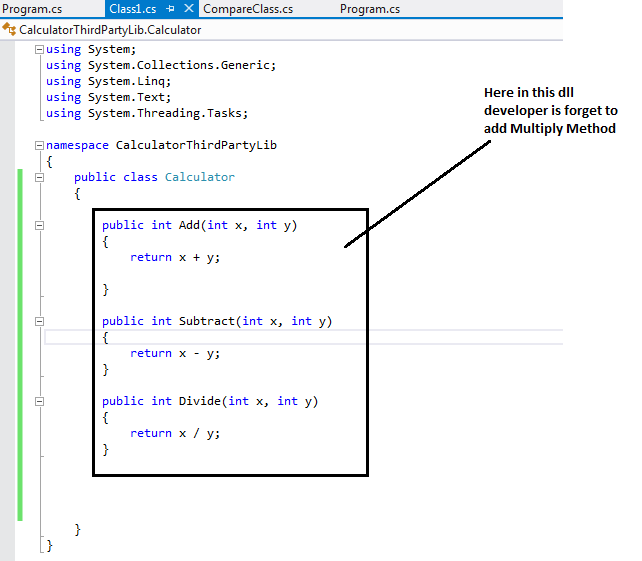##### Code:
`     public class Calculator        {        public int Add(int x, int y)        {            return x + y;        }        public int Subtract(int x, int y)        {            return x - y;        }        public int Divide(int x, int y)        {            return x / y;        }         }`

##### Understanding the code
1. We created class library for calculator
2. Library contains Add, Subtract, Divide methods
3. By mistake developer forget to add Multiply method

1. Consuming Library (Creation of client)

We have added a client (console application to consume this library)1. Adding refrence of Calculator library as created above.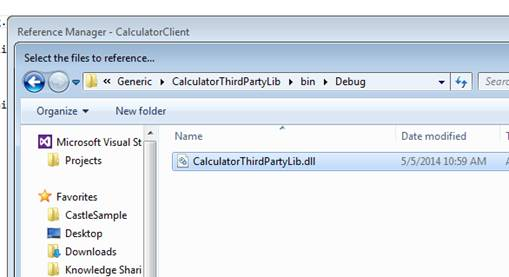>

1. Adding reference to class file as per screen below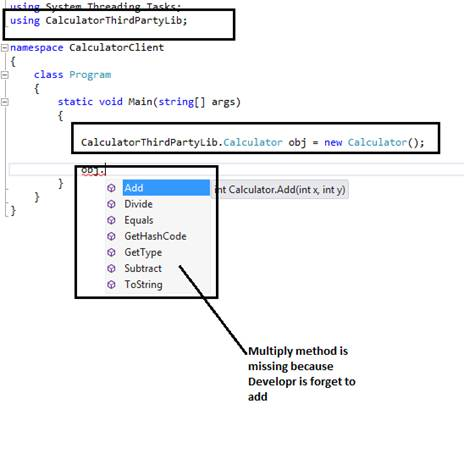Here in code above we have created an object of Calculator class and we can see

here Multiply method is not available because developer forget to add.

So in this situation we have multiple options.

Problem

a.    We can raise a request to Third party dll company to add required

functionality

But it is long process and it would also affect cost and delivery of project because

we have to wait until we get new dll from company

Solution

b.      We can use Extension method to add multiply method of this calculator class

without modifying dll.

This should be static class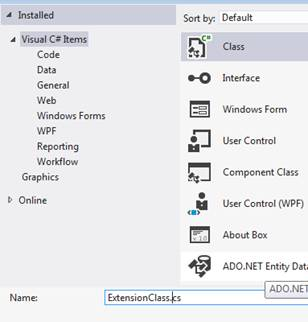1. We added Multiply method as per screen below.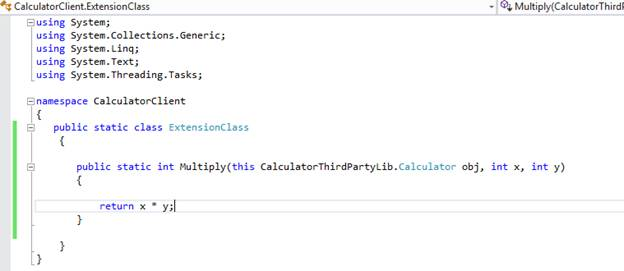##### Sample code:
`namespace CalculatorClient{   public static class ExtensionClass    {       public static int Multiply(this CalculatorThirdPartyLib.Calculator obj, int x, int y)       {           return x * y;       }    }}`
1. Compile code
2. Now as per screen below we can see  Multiply method is now available to

object of calculator class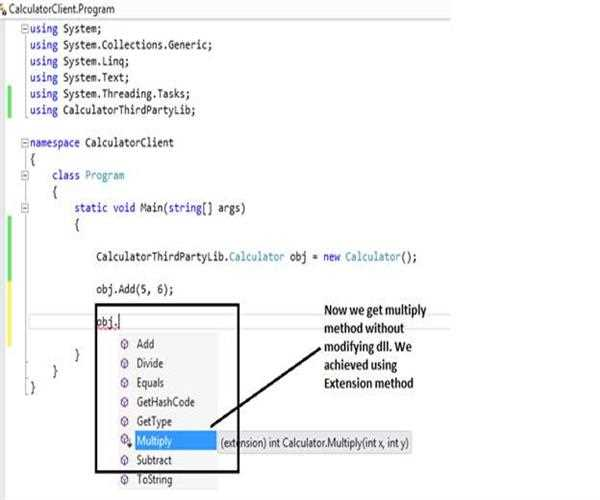>

##### Conclusion

In this article we learned how to create Extension method and why actually we

need it.

Modified On Sep-07-2019 12:14:41 AM